# Energy level facts for kids

Kids Encyclopedia Facts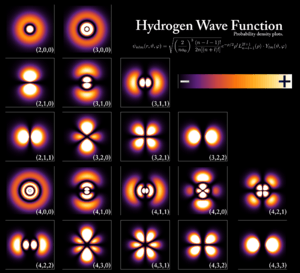Wavefunctions of a hydrogen atom, showing the probability of finding the electron in the space around the nucleus. Each stationary state defines a specific energy level of the atom.

Simply defined as the different states of potential energy for electrons in an atom. A quantum mechanical system can only be in certain states, so that only certain energy levels are possible. The term energy level is most commonly used in reference to the electron configuration in atoms or molecules. In other words, the energy spectrum can be quantized (see continuous spectrum for the more general case).

As with classical potentials, the potential energy is usually set to zero at infinity, leading to a negative potential energy for bound electron states.

Energy levels are said to be degenerate, if the same energy level is obtained by more than one quantum mechanical state. They are then called degenerate energy levels.

The following sections of this article gives an overview over the most important factors that determine the energy levels of atoms and molecules.

## Atoms

### Intrinsic energy levels

#### Orbital state energy level

Assume an electron in a given atomic orbital. The energy of its state is mainly determined by the electrostatic interaction of the (negative) electron with the (positive) nucleus. The energy levels of an electron around a nucleus are given by :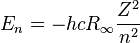$E_n = - h c R_{\infty} \frac{Z^2}{n^2} \$,

where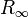$R_{\infty} \$ is the Rydberg constant (typically between 1 eV and 103 eV), Z is the charge of the atom's nucleus,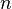$n \$ is the principal quantum number, e is the charge of the electron,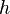$h$ is Planck's constant, and c is the speed of light.

The Rydberg levels depend only on the principal quantum number$n \$.

#### Fine structure splitting

Fine structure arises from relativistic kinetic energy corrections, spin-orbit coupling (an electrodynamic interaction between the electron's spin and motion and the nucleus's electric field) and the Darwin term (contact term interaction of s-shell electrons inside the nucleus). Typical magnitude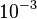$10^{-3}$ eV.

#### Hyperfine structure

Spin-nuclear-spin coupling (see hyperfine structure). Typical magnitude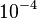$10^{-4}$ eV.

#### Electrostatic interaction of an electron with other electrons

If there is more than one electron around the atom, electron-electron-interactions raise the energy level. These interactions are often neglected if the spatial overlap of the electron wavefunctions is low.

### Energy levels due to external fields

#### Zeeman effect

The interaction energy is: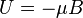$U = - \mu B$ with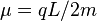$\mu = q L / 2m$

#### Zeeman effect taking spin into account

This takes both the magnetic dipole moment due to the orbital angular momentum and the magnetic momentum arising from the electron spin into account.

Due to relativistic effects (Dirac equation), the magnetic moment arising from the electron spin is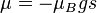$\mu = - \mu_B g s$ with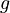$g$ the gyro-magnetic factor (about 2).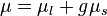$\mu = \mu_l + g \mu_s$ The interaction energy therefore gets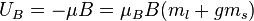$U_B = - \mu B = \mu_B B (m_l + g m_s)$.

#### Stark effect

Interaction with an external electric field (see Stark effect).

## Molecules

Roughly speaking, a molecular energy state, i.e. an eigenstate of the molecular Hamiltonian, is the sum of an electronic, vibrational, rotational, nuclear and translational component, such that: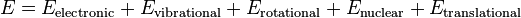$E = E_\mathrm{electronic}+E_\mathrm{vibrational}+E_\mathrm{rotational}+E_\mathrm{nuclear}+E_\mathrm{translational}\,$

where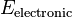$E_\mathrm{electronic}$ is an eigenvalue of the electronic molecular Hamiltonian (the value of the potential energy surface) at the equilibrium geometry of the molecule.

The molecular energy levels are labelled by the molecular term symbols.

The specific energies of these components vary with the specific energy state and the substance.

In molecular physics and quantum chemistry, an energy level is a quantized energy of a bound quantum mechanical state.

## Crystalline Materials

Crystalline materials are often characterized by a number of important energy levels. The most important ones are the top of the valence band, the bottom of the conduction band, the Fermi energy, the vacuum level, and the energy levels of any defect states in the crystals.

## Other pagesEnergy level Facts for Kids. Kiddle Encyclopedia.